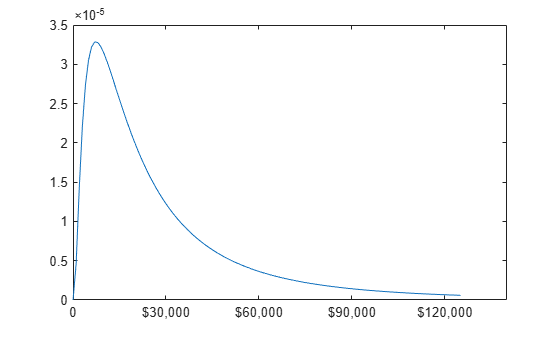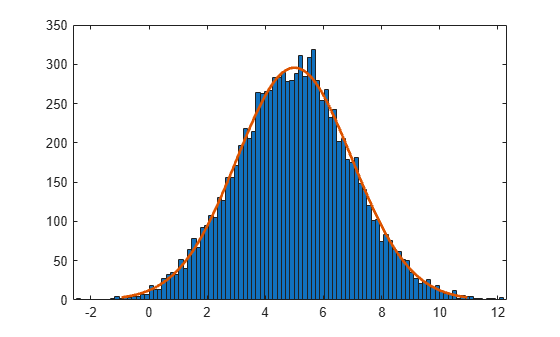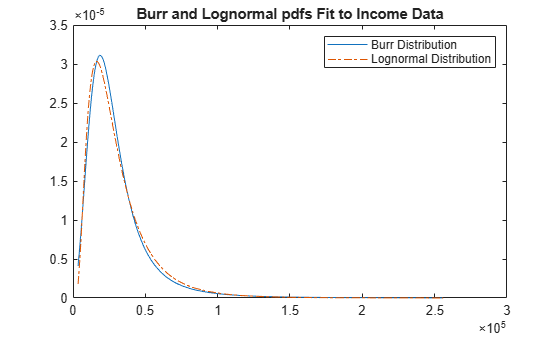Lognormal Distribution

Overview

The lognormal distribution, sometimes called the Galton distribution, is a probability distribution whose logarithm has a normal distribution. The lognormal distribution is applicable when the quantity of interest must be positive, because log(x) exists only when x is positive.

Statistics and Machine Learning Toolbox™ offers several ways to work with the lognormal distribution.

• Create a probability distribution object LognormalDistribution by fitting a probability distribution to sample data (fitdist) or by specifying parameter values (makedist). Then, use object functions to evaluate the distribution, generate random numbers, and so on.

• Work with the lognormal distribution interactively by using the Distribution Fitter app. You can export an object from the app and use the object functions.

• Use distribution-specific functions (logncdf, lognpdf, logninv, lognlike, lognstat, lognfit, lognrnd) with specified distribution parameters. The distribution-specific functions can accept parameters of multiple lognormal distributions.

• Use generic distribution functions (cdf, icdf, pdf, random) with a specified distribution name ('Lognormal') and parameters.

Parameters

The lognormal distribution uses these parameters.

ParameterDescriptionSupport
mu (μ)Mean of logarithmic values$-\infty <\mu <\infty$
sigma (σ)Standard deviation of logarithmic values$\sigma \ge 0$

If X follows the lognormal distribution with parameters µ and σ, then log(X) follows the normal distribution with mean µ and standard deviation σ.

Parameter Estimation

To fit the lognormal distribution to data and find the parameter estimates, use lognfit, fitdist, or mle.

• For uncensored data, lognfit and fitdist find the unbiased estimates of the distribution parameters, and mle finds the maximum likelihood estimates.

• For censored data, lognfit, fitdist, and mle find the maximum likelihood estimates.

Unlike lognfit and mle, which return parameter estimates, fitdist returns the fitted probability distribution object LognormalDistribution. The object properties mu and sigma store the parameter estimates.

Descriptive Statistics

The mean m and variance v of a lognormal random variable are functions of the lognormal distribution parameters µ and σ:

$\begin{array}{l}m=\mathrm{exp}\left(\mu +{\sigma }^{2}/2\right)\\ v=\mathrm{exp}\left(2\mu +{\sigma }^{2}\right)\left(\mathrm{exp}\left({\sigma }^{2}\right)-1\right)\end{array}$

Also, you can compute the lognormal distribution parameters µ and σ from the mean m and variance v:

$\begin{array}{l}\mu =\mathrm{log}\left({m}^{2}/\sqrt{v+{m}^{2}}\right)\\ \sigma =\sqrt{\mathrm{log}\left(v/{m}^{2}+1\right)}\end{array}$

Probability Density Function

The probability density function (pdf) of the lognormal distribution is

$y=f\left(x|\mu ,\sigma \right)=\frac{1}{x\sigma \sqrt{2\pi }}\mathrm{exp}\left\{\frac{-{\left(\mathrm{log}x-\mu \right)}^{2}}{2{\sigma }^{2}}\right\},\text{ }\text{for}\text{\hspace{0.17em}}x>0.$

For an example, see Compute Lognormal Distribution pdf.

Cumulative Distribution Function

The cumulative distribution function (cdf) of the lognormal distribution is

$p=F\left(x|\mu ,\sigma \right)=\frac{1}{\sigma \sqrt{2\pi }}{\int }_{0}^{x}\frac{1}{t}\mathrm{exp}\left\{\frac{-{\left(\mathrm{log}t-\mu \right)}^{2}}{2{\sigma }^{2}}\right\}dt,\text{ }\text{for}\text{\hspace{0.17em}}x>0.$

For an example, see Compute Lognormal Distribution cdf.

Examples

Compute Lognormal Distribution pdf

Suppose the income of a family of four in the United States follows a lognormal distribution with mu = log(20,000) and sigma = 1. Compute and plot the income density.

Create a lognormal distribution object by specifying the parameter values.

pd = makedist('Lognormal','mu',log(20000),'sigma',1)
pd =
LognormalDistribution

Lognormal distribution
mu = 9.90349
sigma =       1

Compute the pdf values.

x = (10:1000:125010)';
y = pdf(pd,x);

Plot the pdf.

plot(x,y)
h = gca;
h.XTick = [0 30000 60000 90000 120000];
h.XTickLabel = {'0','\$30,000','\$60,000',...
'\$90,000','\$120,000'};Compute Lognormal Distribution cdf

Compute the cdf values evaluated at the values in x for the lognormal distribution with mean mu and standard deviation sigma.

x = 0:0.2:10;
mu = 0;
sigma = 1;
p = logncdf(x,mu,sigma);

Plot the cdf.

plot(x,p)
grid on
xlabel('x')
ylabel('p')Relationship Between Normal and Lognormal Distributions

If X follows the lognormal distribution with parameters µ and σ, then log(X) follows the normal distribution with mean µ and standard deviation σ. Use distribution objects to inspect the relationship between normal and lognormal distributions.

Create a lognormal distribution object by specifying the parameter values.

pd = makedist('Lognormal','mu',5,'sigma',2)
pd =
LognormalDistribution

Lognormal distribution
mu = 5
sigma = 2

Compute the mean of the lognormal distribution.

mean(pd)
ans = 1.0966e+03

The mean of the lognormal distribution is not equal to the mu parameter. The mean of the logarithmic values is equal to mu. Confirm this relationship by generating random numbers.

Generate random numbers from the lognormal distribution and compute their log values.

rng('default');  % For reproducibility
x = random(pd,10000,1);
logx = log(x);

Compute the mean of the logarithmic values.

m = mean(logx)
m = 5.0033

The mean of the log of x is close to the mu parameter of x, because x has a lognormal distribution.

Construct a histogram of logx with a normal distribution fit.

histfit(logx)The plot shows that the log values of x are normally distributed.

histfit uses fitdist to fit a distribution to data. Use fitdist to obtain parameters used in fitting.

pd_normal = fitdist(logx,'Normal')
pd_normal =
NormalDistribution

Normal distribution
mu = 5.00332   [4.96445, 5.04219]
sigma = 1.98296   [1.95585, 2.01083]

The estimated normal distribution parameters are close to the lognormal distribution parameters 5 and 2.

Compare Lognormal and Burr Distribution pdfs

Compare the lognormal pdf to the Burr pdf using income data generated from a lognormal distribution.

Generate the income data.

rng('default') % For reproducibility
y = random('Lognormal',log(25000),0.65,[500,1]);

Fit a Burr distribution.

pd = fitdist(y,'burr')
pd =
BurrDistribution

Burr distribution
alpha = 26007.2   [21165.5, 31956.4]
c = 2.63743   [2.3053, 3.0174]
k = 1.09658   [0.775479, 1.55064]

Plot both the Burr and lognormal pdfs of income data on the same figure.

p_burr = pdf(pd,sortrows(y));
p_lognormal = pdf('Lognormal',sortrows(y),log(25000),0.65);
plot(sortrows(y),p_burr,'-',sortrows(y),p_lognormal,'-.')
title('Burr and Lognormal pdfs Fit to Income Data')
legend('Burr Distribution','Lognormal Distribution')Related Distributions

 Abramowitz, Milton, and Irene A. Stegun, eds. Handbook of Mathematical Functions: With Formulas, Graphs, and Mathematical Tables. 9. Dover print.; [Nachdr. der Ausg. von 1972]. Dover Books on Mathematics. New York, NY: Dover Publ, 2013.

 Evans, M., N. Hastings, and B. Peacock. Statistical Distributions. 2nd ed., Hoboken, NJ: John Wiley & Sons, Inc., 1993.

 Lawless, J. F. Statistical Models and Methods for Lifetime Data. Hoboken, NJ: Wiley-Interscience, 1982.

 Marsaglia, G., and W. W. Tsang. “A Fast, Easily Implemented Method for Sampling from Decreasing or Symmetric Unimodal Density Functions.” SIAM Journal on Scientific and Statistical Computing. Vol. 5, Number 2, 1984, pp. 349–359.

 Meeker, W. Q., and L. A. Escobar. Statistical Methods for Reliability Data. Hoboken, NJ: John Wiley & Sons, Inc., 1998.

 Mood, A. M., F. A. Graybill, and D. C. Boes. Introduction to the Theory of Statistics. 3rd ed., New York: McGraw-Hill, 1974. pp. 540–541.